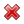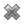# Fight Finance

#### CoursesTagsRandomAllRecentScoresScoreskeithphw $6,011.61 Jade$1,815.80 Chu $789.98 royal ne...$750.00 Leehy $713.33 Visitor$650.00 JennyLI $625.61 Visitor$590.00 ZOE HY $560.00 Visitor$555.33 Visitor $550.00 Visitor$550.00 Visitor $540.00 Visitor$500.00 Yizhou $489.18 Visitor$480.00 Visitor $464.70 Visitor$460.00 Visitor $460.00 LWH$460.00

Below are some statements about futures and European-style options on non-dividend paying stocks. Assume that the risk free rate is always positive. Which of these statements is NOT correct? All other things remaining equal:

Which of the following statements about an option (either a call or put) and its underlying stock is NOT correct?

Which of the following statements about an option (either a call or put) and its underlying stock is NOT correct?

 European Call Option on a non-dividend paying stock Description Symbol Quantity Spot price ($) $S_0$ 20 Strike price ($) $K_T$ 18 Risk free cont. comp. rate (pa) $r$ 0.05 Standard deviation of the stock's cont. comp. returns (pa) $\sigma$ 0.3 Option maturity (years) $T$ 1 Call option price ($) $c_0$ 3.939488 Delta $\Delta = N[d_1]$ 0.747891 $N[d_2]$ $N[d_2]$ 0.643514 Gamma $\Gamma$ 0.053199 Theta ($/year) $\Theta = \partial c / \partial T$ 1.566433